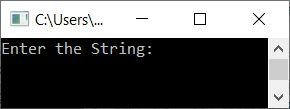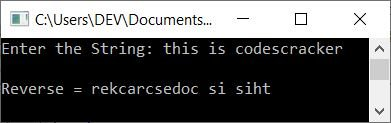# C++ Program to Reverse a String

In this article, you will learn and get code to reverse a string in C++ programming. Here is the list of programs for reversing a string entered by the user at run-time:

## Reverse a string without using a library function

To reverse a string in C++ programming, ask the user to enter a string. Then reverse it and print the reversed string as shown in the program given below:

The question is: write a C++ program to reverse a string entered by the user. Here is its answer:

```#include<iostream>
#include<stdio.h>
using namespace std;
int main()
{
char str, strTemp;
int len, i=0;
cout<<"Enter the String: ";
gets(str);
while(str[i]!='\0')
i++;
len = i;
strTemp[len] = '\0';
len--;
i = 0;
while(str[i]!='\0')
{
strTemp[len] = str[i];
i++;
len--;
}
i=0;
while(strTemp[i]!='\0')
{
str[i] = strTemp[i];
i++;
}
cout<<"\nReverse = "<<str;
cout<<endl;
return 0;
}```

This program was built and runs under the Code::Blocks IDE. Here is its sample run:Now, as input, enter the string "this is codescracker" and press the ENTER key to reverse it and print its reverse, as shown in the screenshot below:The following block of code:

```while(str[i]!='\0')
i++;```

is used to find the length of a string. That is, if a string says "this is codescracker" entered by the user, then it gets stored in str in a way that:

• str=t
• str=h
• str=i
• and so on up until
• str=r

And at the last index, a null-terminated character (\0) automatically gets assigned. So str=\0.

Because the initial value of i is 0, the while loop condition str[i]!='\0' or str!='\0' or t!='\0' evaluates to true, and the value of i is incremented and the condition is evaluated again. This process continues until the condition is evaluated as false. When the condition gets evaluated to be false, then the variable i holds its value of 20, which is the length of the entered string.

The following block of C++ code:

```while(str[i]!='\0')
{
strTemp[len] = str[i];
i++;
len--;
}```

is used to copy the original string (in character-by-character mode) in a way that the character at the first (0th) index of str gets initialized to the last index of strTemp. That is, we copied the original string into strTemp[] in a way that strTemp holds the original string in reverse order.

And using this block of code:

```while(strTemp[i]!='\0')
{
str[i] = strTemp[i];
i++;
}```

The value of strTemp gets copied to str. Because strTemp holds the original string's value in reverse order, the reverse of the string gets initialized to str in a character-by-character manner. Now print the value of str on the output, which shows the reverse of the entered string.

#### Reverse a string without using another string

Previous programs can also be made in this manner without the use of any other character array (string) such as strTemp[]:

```#include<iostream>
#include<stdio.h>
using namespace std;
int main()
{
char str, ch;
int len, i=0, j;
cout<<"Enter the String: ";
gets(str);
while(str[i]!='\0')
i++;
len = i;
i = 0;
j = len-1;
while(i<j)
{
ch = str[i];
str[i] = str[j];
str[j] = ch;
i++;
j--;
}
cout<<"\nReverse = "<<str;
cout<<endl;
return 0;
}```

This program works by moving characters from the 0th index to the last index, and characters from the last index to the 0th index. Again, the character at the second index gets moved to the second last index, and the character at the second last index gets moved to the second index, and so on.

Here the variable i starts from 0, whereas the variable j starts from len-1 (or the last index's value). And the process of moving the characters continues until the value of i becomes equal to or greater than the value of j.

## Reverse a string using a while loop

This program reverse a string using a while loop. It is exactly the same program as the previous one, except this program uses the strlen() function of the string.h header file to find the length of a string.

```#include<iostream>
#include<stdio.h>
#include<string.h>
using namespace std;
int main()
{
char str, ch;
int i, j, len;
cout<<"Enter the String: ";
gets(str);
i = 0;
len = strlen(str);
j = len-1;
while(i<j)
{
ch = str[i];
str[i] = str[j];
str[j] = ch;
i++;
j--;
}
cout<<"\nReverse = "<<str;
cout<<endl;
return 0;
}```

## Reverse a string using a for loop

Now let's create the same program using the for loop. All the programs given here produce the same output:

```#include<iostream>
#include<stdio.h>
#include<string.h>
using namespace std;
int main()
{
char str, ch;
int i, j, len;
cout<<"Enter the String: ";
gets(str);
len = strlen(str);
j = len-1;
for(i=0; i<j; i++, j--)
{
ch = str[i];
str[i] = str[j];
str[j] = ch;
}
cout<<"\nReverse = "<<str;
cout<<endl;
return 0;
}```

#### The same program in different languages

C++ Quiz

« Previous Program Next Program »